# Examples for secondary school students - page 44

1. Probability - tickets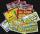What is the probability that when you have 25 tickets in 5000 that you wins the first (one) prize?
2. SalamiHow many ways can we choose 5 pcs of salami, if we have 6 types of salami for 10 pieces and one type for 4 pieces?
3. Vectors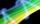For vector w is true: w = 2u-5v. Determine coordinates of vector w if u=(3, -1), v=(12, -10)
4. ThrowWe throw 2 times with 2 dices. What is the probability that the first roll will fall more than sum of 9 and the second throw have sum 3 or does not have the sum 4?
5. Three numbersFind three numbers so that the second number is 4 times greater than the first and the third is lower by 5 than the second number. Their sum is 67.
6. RectanglePerimeter of rectangle is 48 cm. Calculate its dimensions if they are in the ratio 5:3 (width:height)
7. Cube diagonalsDetermine the volume and surface area of the cube if you know the length of the body diagonal u = 216 cm.
8. Cans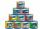How many cans must be put in the bottom row if we want 182 cans arrange in 13 rows above so that each subsequent row has always been one tin less? How many cans will be in the top row?
9. LineIt is true that the lines that do not intersect are parallel?
10. Sinus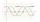Determine the smallest integer p for which the equation 4 sin x = p has no solution.
11. Target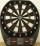Peter, Martin and Jirka were fire in a special target, which had only three fields with values of 12, 18 and 30 points. All boys were firing with the same number of arrows and all the arrows hit the target, and the results of every two boys differed in one
12. SequenceWrite the first 6 members of these sequence: a1 = 5 a2 = 7 an+2 = an+1 +2 an
13. Coffee shop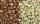To the coffee shop brought 2 types of coffee totally 50 kg. The first type was CZK 220 per kilogram, coffee second type 300 CZK per 1 kg. For all the coffee trader earned CZK 12,000. How many kilograms of coffee of first type and how many kilograms of cof
14. Vector - basic operationsThere are given points A [-9; -2] B [2; 16] C [16; -2] and D [12; 18] a. Determine the coordinates of the vectors u=AB v=CD s=DB b. Calculate the sum of the vectors u + v c. Calculate difference of vectors u-v d. Determine the coordinates of the vector w.
15. Quadrilateral 2Show that the quadrilateral with vertices P1(0,1), P2(4,2) P3(3,6) P4(-5,4) has two right triangles.
16. Right angled triangleHypotenuse of a right triangle is 17 cm long. When we decrease length of legs by 3 cm then decrease its hypotenuse by 4 cm. Determine the size of legs.
17. SinesIn ▵ ABC, if sin(α)=0.5 and sin(β)=0.6 calculate sin(γ)
18. Three dice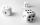When you throw three dice was the sum total of the dice 10. The yellow dice fell one eye more than on the red and brown fell 3 eyes less than red. How many eyes fell on every dice?
19. Cubes - diffSecond cubes edge is 2 cm longer than the edge of the first cube. Volume difference blocks is 728 cm3. Calculate the sizes of the edges of the two dice.
20. Log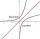Calculate value of expression log |3 +7i +5i2| .

Do you have an interesting mathematical example that you can't solve it? Enter it, and we can try to solve it.

To this e-mail address, we will reply solution; solved examples are also published here. Please enter e-mail correctly and check whether you don't have a full mailbox.